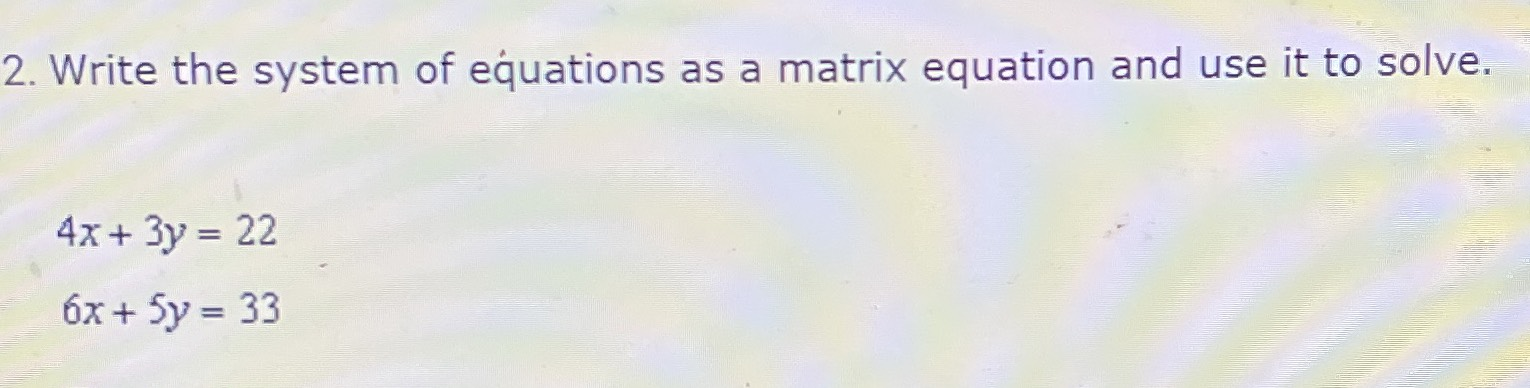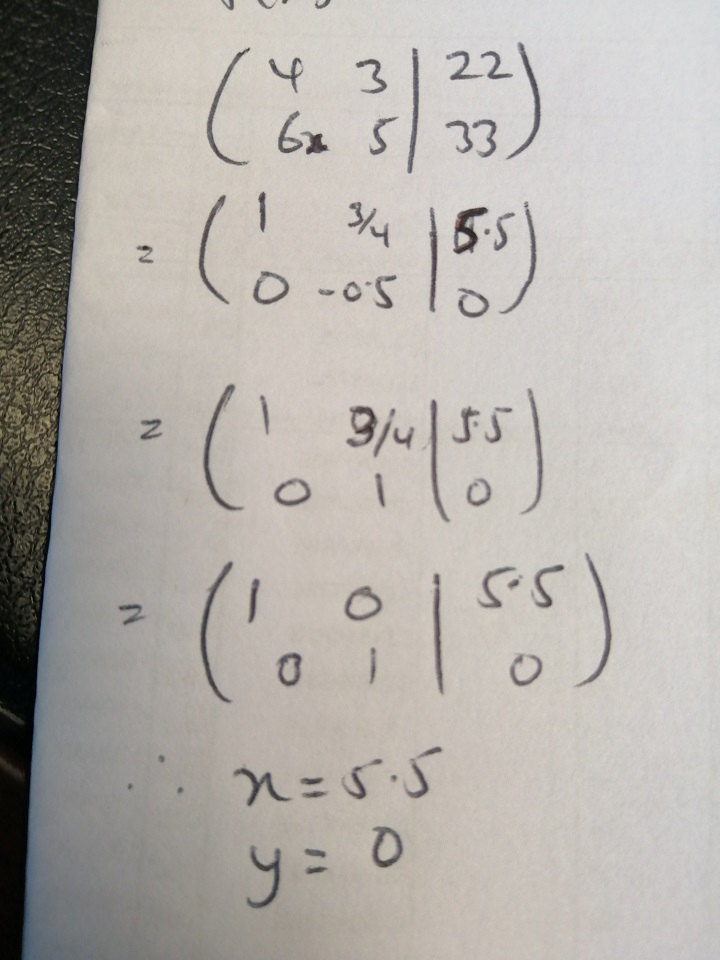### ¿Todavía tienes preguntas de matemáticas?

Pregunte a nuestros tutores expertos
Algebra
Pregunta2. Write the system of equations as a matrix equation and use it to solve.

$$4 x + 3 y = 22$$

$$6 x + 5 y = 33$$